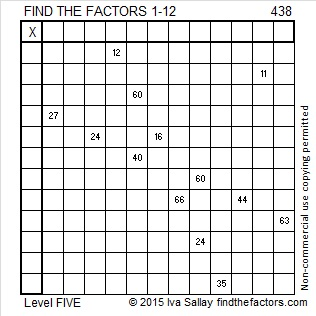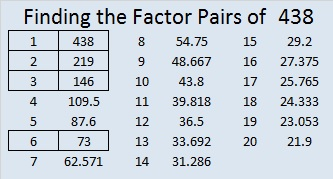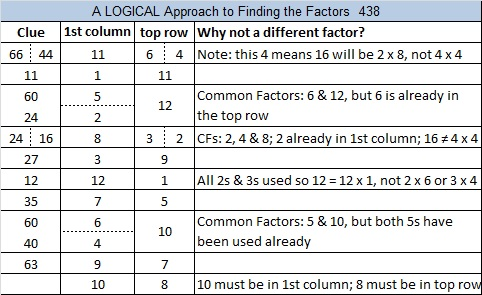# 438 and Level 5

The sum of the digits of 438 is 4 + 3 + 8 = 15. The sum of the digits of its prime factorization (2 x 3 x 73) is 2 + 3 + 7 + 3 = 15. Since the two sums are equal, 438 is the 18th Smith number.Print the puzzles or type the factors on this excel file: 12 Factors 2015-03-23

• 438 is a composite number.
• Prime factorization: 438 = 2 x 3 x 73
• The exponents in the prime factorization are 1, 1, and 1. Adding one to each and multiplying we get (1 + 1)(1 + 1)(1 + 1) = 2 x 2 x 2 = 8. Therefore 438 has exactly 8 factors.
• Factors of 438: 1, 2, 3, 6, 73, 146, 219, 438
• Factor pairs: 438 = 1 x 438, 2 x 219, 3 x 146, or 6 x 73
• 438 has no square factors that allow its square root to be simplified. √438 ≈ 20.9284